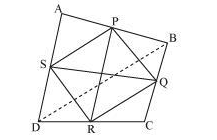# Prove that the line segments joining the midpoints of opposite sides of a quadrilateral bisect each other.

Question:

Prove that the line segments joining the midpoints of opposite sides of a quadrilateral bisect each other.

Solution:

Let ABCD be the quadrilateral in which P, Q, R, and S are the midpoints of sides AB, BC, CD, and DA, respectively.
Join PQ, QR, RS, SP and BDBD is a diagonal of ABCD.In ΔABDS and P are the midpoints of AD and AB, respectively.

$\therefore S P \| B D$ and $S P=\frac{1}{2} B D$.  ... (i)        (By midpoint theorem)

Similarly in Δ BCD, we have:

$Q R \| B D$ and $Q R=\frac{1}{2} B D$   ... (ii)   (By midpoint theorem)

From equations (i) and (ii), we get:
SP ||  BD || QR

∴ SP || QR and SP = QR  [Each equal to $\frac{1}{2}$ BD]

In quadrilateral SPQR, one pair of the opposite sides is equal and parallel to each other.

∴  SPQR is a parallelogram.

We know that the diagonals of a parallelogram bisect each other.

∴ PR and QS bisect each other.